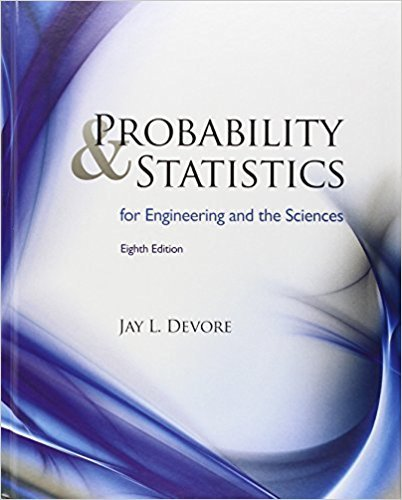×
Get Full Access to Probability And Statistics For Engineering And The Sciences - 8 Edition - Chapter 1 - Problem 48e
Get Full Access to Probability And Statistics For Engineering And The Sciences - 8 Edition - Chapter 1 - Problem 48e

×

# Solved: Exercise 34 presented the following data onISBN: 9780538733526 61

## Solution for problem 48E Chapter 1

Probability and Statistics for Engineering and the Sciences | 8th Edition

• Textbook Solutions
• 2901 Step-by-step solutions solved by professors and subject experts
• Get 24/7 help from StudySoup virtual teaching assistantsProbability and Statistics for Engineering and the Sciences | 8th Edition

4 5 1 362 Reviews
10
0
Problem 48E

Problem 48E

Exercise 34 presented the following data on endotoxin concentration in settled dust both for a sample of urban homes and for a sample of farm homes:a. Determine the value of the sample standard deviation for each sample, interpret these values, and then contrast variability in the two samples. [Hint:∑xi=237.0 for the urban sample and =128.4 for the farm sample, and ∑x2i = 10,079 for the urban sample and 1617.94 for the farm sample.]

b. Compute the fourth spread for each sample and compare. Do the fourth spreads convey the same message about variability that the standard deviations do? Explain.

c. The authors of the cited article also provided endotoxin concentrations in dust bag dust:Construct a comparative boxplot (as did the cited paper) and compare and contrast the four samples.

Step-by-Step Solution:
Step 1 of 3

Biol 490 Cancer Biology Section 54 (on-line) Dr. Meerschaert WSB 220 jmeerschaert@stcloudstate.edu This course is being offered as a 3 credit elective in the Biomedical Sciences major. As a temporary course number it may need to be added to your elective list after completion of the course by your major advisor. This course will cover the basics of how cells become altered. Some basic cell biology will be needed to fully understand all topics. If you have limited background in biology and need help understanding anything covered in this course, please contact me using the e-mail address given above. st Required text: Principles of Cancer Biology by Lewis J. Kleinsmith. 1 edition, 2006. ISBN: 0-8053-4003-3 Published

Step 2 of 3

Step 3 of 3

##### ISBN: 9780538733526

Since the solution to 48E from 1 chapter was answered, more than 319 students have viewed the full step-by-step answer. The full step-by-step solution to problem: 48E from chapter: 1 was answered by , our top Statistics solution expert on 08/08/17, 06:52AM. The answer to “Exercise 34 presented the following data on endotoxin concentration in settled dust both for a sample of urban homes and for a sample of farm homes: a. Determine the value of the sample standard deviation for each sample, interpret these values, and then contrast variability in the two samples. [Hint:?xi=237.0 for the urban sample and =128.4 for the farm sample, and ?x2i = 10,079 for the urban sample and 1617.94 for the farm sample.]b. Compute the fourth spread for each sample and compare. Do the fourth spreads convey the same message about variability that the standard deviations do? Explain.c. The authors of the cited article also provided endotoxin concentrations in dust bag dust: Construct a comparative boxplot (as did the cited paper) and compare and contrast the four samples.” is broken down into a number of easy to follow steps, and 129 words. This textbook survival guide was created for the textbook: Probability and Statistics for Engineering and the Sciences , edition: 8. Probability and Statistics for Engineering and the Sciences was written by and is associated to the ISBN: 9780538733526. This full solution covers the following key subjects: sample, farm, dust, Urban, cited. This expansive textbook survival guide covers 16 chapters, and 1314 solutions.

Unlock Textbook Solution